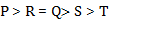# Reasoning Quiz for RRB PO & SBI CLERK MAINS 2019 | 8 July 2019

## Reasoning Quiz for RRB PO & SBI CLERK MAINS

Reasoning Quiz to improve your  Reasoning  for SBI Po & SBI clerk exam Reasoning, IBPS PO Reasoning , IBPS Clerk Reasoning , IBPS RRB Reasoning, LIC AAO , LIC ADO,  and other competitive exams.

Q1. M, T, B, D and R are five friends each of them having a different weight. R is heavier than M and T but lighter than only B. M is heavier than only T. Who among the following is at the third position according to their weights?

A) R

B) M

C) D

D) T

E) None of these

Q1. Ans(C)

B > R > D > M > T

Q2. Among D, E, F, G and H, each having a different height, F is taller than only D and G is shorter than E and taller than H. Who among the following is the tallest?

A) E

B) H

C) G

D) F

E) None of these

Q2. Ans(A)

E > G > H > F > D

Q3. Jayant is taller than Rajeev but not as tall as Madhu. Madhu is not as tall as Pranav. Who is the tallest among Rajeev, Madhu, Jayant and Pranav ?

A) Rajeev

C) Jayant

D) Pranav

E) Can’t be determined.

Q3. Ans(D)

Pranav > Madhu > Jayant > Rajeev

Q4. Raghav is twenty first from the left end in a row of 40 boys, and Prarthi is twenty first from the right end in the same row. How many boys are there between them in the row ?

A) One

B) Two

C)  Three

E) None of these

Q4. Ans(E)Q5. P is greater than R. Q is greater than S, who is greater than T. Q is equal to R. than who is greatest?

A) P

B) Q

C) R

D) S

E) T

Q5. Ans(A)Direction (6-10): The following questions are based on the five three – digit numbers given below:
547   247    465    742    343
Q6. If 3 is added to the second digit of each of the numbers how many numbers thus formed will be divisible by three?
(a) None
(b) One
(c) Two
(d) Three
(e) None of these
Q6. Ans.(b)

Only 465 will be divisible by 3 when added 3 to the second digit of each number.
Q7. If all the digits in each of the numbers are arranged in descending order within the number, which of the following will be the highest number in the new arrangement of numbers?
(a) 547
(b) 247
(c) 465
(d) 742
(e) 343
Q7. Ans.(a)
754    742     654     742    433
Q8. What will be the resultant number if the second digit of the second lowest number is divided by the third digit of the highest number?
(a) 2
(b) 3
(c) 0
(d) 1
(e) 4
Q8. Ans.(a)
4÷2=2
Q9. If 3 is added to the first digit and 1 is added to the last digit of each of the numbers then which of the following numbers will be the second highest number?
(a) 547
(b) 247
(c) 465
(d) 742
(e) 343
Q9. Ans.(a)
848    548    766   1043   644
Q10. If in each number the first and the third digits are interchanged then which will be the highest number?
(a) 547
(b) 247
(c) 465
(d) 742
(e) 343
Q10. Ans.(a)

745   742    564    247    343

### You Can Read This Also:

Best E-books for LIC AAO 2019 : Get PDF here

ambitiousbaba.com need your support to Grow

I challenge you will get Best Content in Our PDFs with Detail solutions and Latest Pattern

Memory Based Puzzle E-book | 2016-19 Exams Covered

Get PDF here

Caselet Data Interpretation 200 Questions

Get PDF here
Puzzle & Seating Arrangement E-Book for BANK PO MAINS (Vol-1)

Get PDF here

### ARITHMETIC DATA INTERPRETATION 2019 E-book

Get PDF here
The Banking Awareness 500 MCQs E-book| Bilingual (Hindi + English)

Get PDF here

High Level DATA INTERPRETATION Practice E-BOOK

Get PDF here

### How to Access on App:-

1. Go to Playstore search Ambitious Baba or Click here to Install App